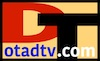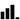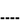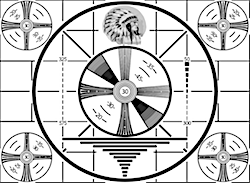Blog# TV Minimum SignalSignals just below the TV's required minimum the picture starts to pixelate, and disappears only a few decibels (dB's) lower. Signal power is measured on a base 10 logarithmic scale in dBm -- decibels (dB's) above or below 1 milliwatt.

Televisions will process signal power levels between a high of - 5 dBm to as low as around -65 dBm. Above -5 dBm the receiver is overwhelmed and cannot process the signal. The required minimum signal mainly depends on local conditions (electronic noise pollution), and the receiver sensitivity. Receiver sensitivity depends on its' Noise Figure and minimum Signal-to-Noise ratio. Under ideal conditions (TV antenna > 30 feet high, no electronic pollution, and a hightly sensitive receiver) a TV might get a -80 dBm signal. Most televisions and conditions will require a minimum signal around -65 dBm.

TV SIGNAL Power (dBm) Percent-30 to -5 58 - 100%-45 to -30 33 - 58%-55 to -45 17 - 33%-65 to -55 0 - 17%Low S/N -75 to -65

ESTIMATE a TV's MINIMUM SIGNAL
Noise Figure (NF) and Signal-to-Noise (S/N) ratio are the primary factors that determine sensitivity. Noise Figure is a measure of receiver noise, the noise the receiver adds to the signal. The lower the NF the better. The minimum Signal-to-Noise (S/N) ratio is the difference between the signal and average noise level (signal greater than noise). The lower the required S/N the better.

Specification Typical Variation
Noise Figure (NF) 10 dB 6 to 15 dB
Signal-to-Noise (S/N) 30 dB 20 to 35 dB

Television receivers typically have a noise figure of 10 dB, but it can be as low as 6 dB, to as high as 15 dB or more. A minimum signal-to-noise ratio of 30 dB is required in most cases due to interference from other signals, multi-path, and electronic noise.

Another factor is background noise, also called thermal or kTB noise, and is based on receiver noise bandwidth (6 MHz for an RF channel), and temperature. Temperature has a minor effect, and it is standard practice to use 300°K (80°F / 27°C), or 290°K (62°F / 17°C) for calculations. Noise is random with an average deviation of about ± 5 to ± 10 dB from the average level.

 Pmin = kTB (NF) (S/N) Pmin = Minimum Signal (watts). k = Boltzmann's Constant= 1.380 622 x 10-23 (Watt sec / °K). T = Temperature in Degrees Kelvin (°K). B = Receiver Noise Bandwidth in Hertz (Hz). = 6,000,000 Hz (6 MHz) for an RF channel. NF - Noise Figure (ratio). S/N - Minimum Signal-to-Noise (ratio).Early Test Pattern
 Estimate TV Minimum Signal Noise Figure (NF): dB Signal-to-Noise (S/N): dB Bandwidth (B): MHz Temperature (T): °K °F °C Bandwidth Temperature Noise Floor (kTB) + Noise Factor (NF) = Noise out of Receiver (kTB NF) + Minimum Signal-to-Noise: Minimum TV Signal (dBm)OTA DTv TV Minimum Signal